Refer to our Texas Go Math Grade 4 Answer Key Pdf to score good marks in the exams. Test yourself by practicing the problems from Texas Go Math Grade 4 Module 10 Assessment Answer Key.

Vocabulary

compatible numbers
partial quotient

Vocabulary

Choose the best term from the box to complete the sentence.

Question 1.
You use the _____________ method of dividing when multiples of the divisor are subtracted from the dividend and then the quotients are added together.

You use the partial quotient  method of dividing when multiples of the divisor are subtracted from the dividend and then the quotients are added together.

Concepts and Skills

Use repeated subtraction to divide.

Question 2.
78 ÷ 6 = ____________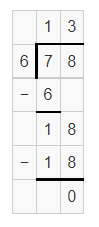Quotient : 13

Remainder: 0

Question 3.
57 ÷ 3 = ___________Quotient : 19

Remainder : 0

Question 4.
98 ÷ 7 = __________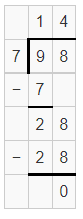Quotient :14

Remainder : 0

Divide. Use partial quotients.

Question 5.
184 ÷ 8 = __________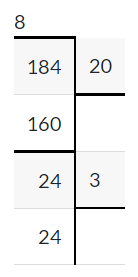Quotients : 20+3 = 23

Remainder : 0

Question 6.
276 ÷ 6 = __________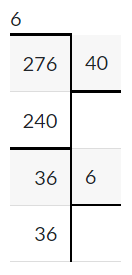Quotient : 40+6

Remainder : 0

Question 7.
742 ÷ 7 = __________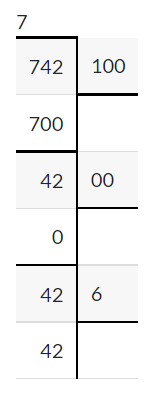On adding partial quotients : 100+6 = 106

Remainder: 0

Question 8.
552 ÷ 4 = ____________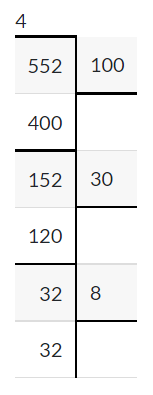On adding partial quotients : 100+30+8

Remainder : 0

Divide.

Question 9.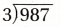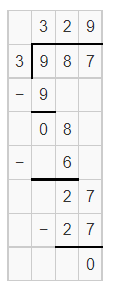Quotient : 329

Remainder : 0

Question 10.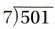Quotient : 071

Remainder : 4

Question 11.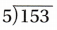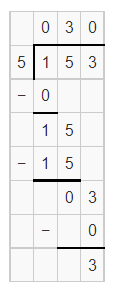Quotient : 030

Remainder : 3

Question 12.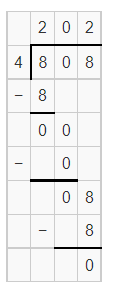Quotient : 202

Remainder : 0

Question 13.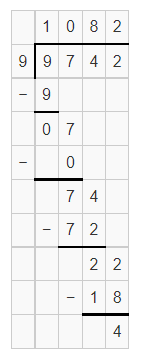Quotient: 1087

Remainder :4

Question 14.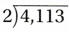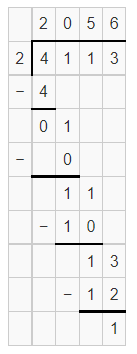Quotient : 2056

Remainder : 1

Question 15.
Mrs. Valdez bought 6 boxes of roses. Each box had 24 roses. She divided all the roses into 9 equal-sized bunches. How many roses were in each bunch?
(A) 54
(B) 16
(C) 26
(D) 18

Number of boxes = 6

Number of roses in each box = 24

Total number of roses in 6 boxes = 144

Also given, She divided all the roses into 9 equal-sized bunches.

So, 144/9 = 16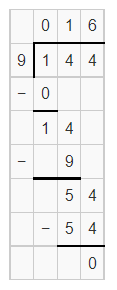There are 16 roses in each bunch.

Question 16.
There are 152 students playing instruments in the marching band and 24 students carry flags. All of the students are arranged in equal rows of 8 students for a parade. How many rows of students are there?
(A)22 rows
(B) 220 rows
(C) 21 rows
(D) 120 rows

Number of students playing instruments = 152

Number of students carrying flag = 24

The number of rows students were arranged = 8

So, Total number of students = 152 + 24 = 176

Now, The number of students of rows =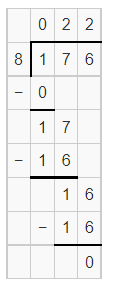The number of rows of students = 22

Question 17.
A trucking company used 8 trucks to ship an order of 820 boxes and another order of 428 boxes. The 8 trucks were each loaded with an equal number of boxes. How many boxes were loaded onto each truck?

Record your answer and fill in the bubbles on the grid. Be sure to use the correct place value.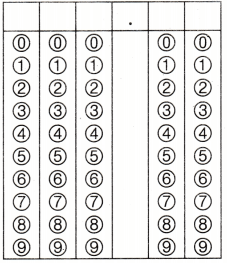Number of trucks = 8

Number of boxes company shipped = 820

Number of boxes another order shipped = 428

Total number of boxes = 1,248

Now, The number of boxes were loaded in each truck =Therefore, the number of boxes loaded in to each truck = 156.

Scroll to Top